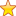# Round Robin Scheduling Algorithm in Operating System (OS)

## Round Robin Scheduling Algorithm in Operating System

In Round robin Scheduling Algorithm, each process is given a fixed time called quantum for execution. After the Quantum of time passes, the current running process is preempted and the next process gets executed for next quantum of time.

CPU Scheduler goes around the ready queue and allocates CPU to each process for the interval upto 1 time quantum. Ready Queue is like a First In First Out Structure, where new processes are at last of the ready queue.

Now, while a process is in that quantum executing, one of the two things will happen

1. If the process has a CPU burst of less than quantum time, then as the process gets finished , CPU resources are released voluntarily. the next process in the ready queue occupies the CPU.
2. If the CPU burst is larger than the quantum, the timer will go off and the process is preempted, its state is saved by context switching and process is put at last of ready queue. After this, the next process in the ready queue comes in CPU quantum.

### Round Robin Example

Let’s understand round Robin Scheduling with the help of an example .

ProcessBurst Time
P110
P22
P35
P46

The order in which the CPU processes the process are (Gantt Chart) –

Here, we have taken quantum as 3ms. So,each process is assigned 3ms before switching to next process.

• P1 is executed for 3ms. The, P2 is executed. As, P2 is only 2ms long, So after 2 ms , next process occupies the CPU.
• P3 then is executed for 3ms. So, P3 remaining = 2ms. Then , P4 is executed for 3ms. P4 remaining time = 3ms
• P1 is again executed for 3ms. P1 remaining time = 4ms
• P3 is executed for 2ms. Then, P4 is executed for 3ms.
• Now remaining process is P1 only . It executed for 3 ms. After that, Since P2,P3 and P4 are already finished, P1 is executed again for remaining time (1ms).Let’s calculate Average Waiting time for each process –

• Average waiting time for P1 = 19- (3+3) = 13ms
• Average Waiting time for P2 = 3ms
• Average Waiting time for P3 = 14-3 = 11ms
• Average Waiting time for P4 = 16-3 = 13ms

Total average waiting time = (13 +3 +11+13)/4 = 10ms

• It does not cause starvation as all process get equal time of CPU.5